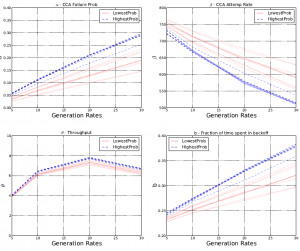# Case Study: WSN Term Project at IIT-M with NetSim

Wireless Sensor Networks (WSN) is an active area of research and given below is a case study of a WSN term project done at Indian Institute of Technology (IIT) Madras using NetSim, titled “Estimation of Carrier Sense Range and its effect on IEEE 802.15.4 Sensor Networks”

Challenge

• Building probability models: The attenuation of IEEE 802.15.4 signals was to be observed and probability models for decoding range and carrier sense range were to be developed.
• Estimating decoding range: This is the threshold below which packets sent from the transmitter are always received, and was to be estimated in terms of the probability that two devices separated by a distance are in decoding range.
Use Case
• Developing custom code: Source C files, Sensor.c, CheckRoute.c and CCA.c of NetSim WSN library were modified. These codes were linked and debugged using NetSim’s project work environment.
• Modeling network configurations: Custom Sensor network configurations were created using NetSim’s XML based network configuration files
• Generating performance metrics: Packet and event traces were used for analyzing network performance metrics such as Throughput, Probability of packet discard, Average service rate and Mean Backoff duration
Results
• Creating dependency graphs• These are directed graphs representing the probability of link establishment between sensors.These graphs were generated by sampling the probability model for each link.
• Statistically analyzing performance: Variation in network performance, from simulation, for different dependency graphs were plotted for the 10 most likely and 10 least likely DG’s
• Establishing confidence interval: This provides the probability that a network will operate in a corresponding range. The confidence interval for operation of the 802.15.4 Wireless Sensor network with limits of performance, i.e. throughput and packet failure probability, was established.
The complete report (1.3 Mb) is available at http://tetcos.com/WSN_Project_Report_IITM.pdf